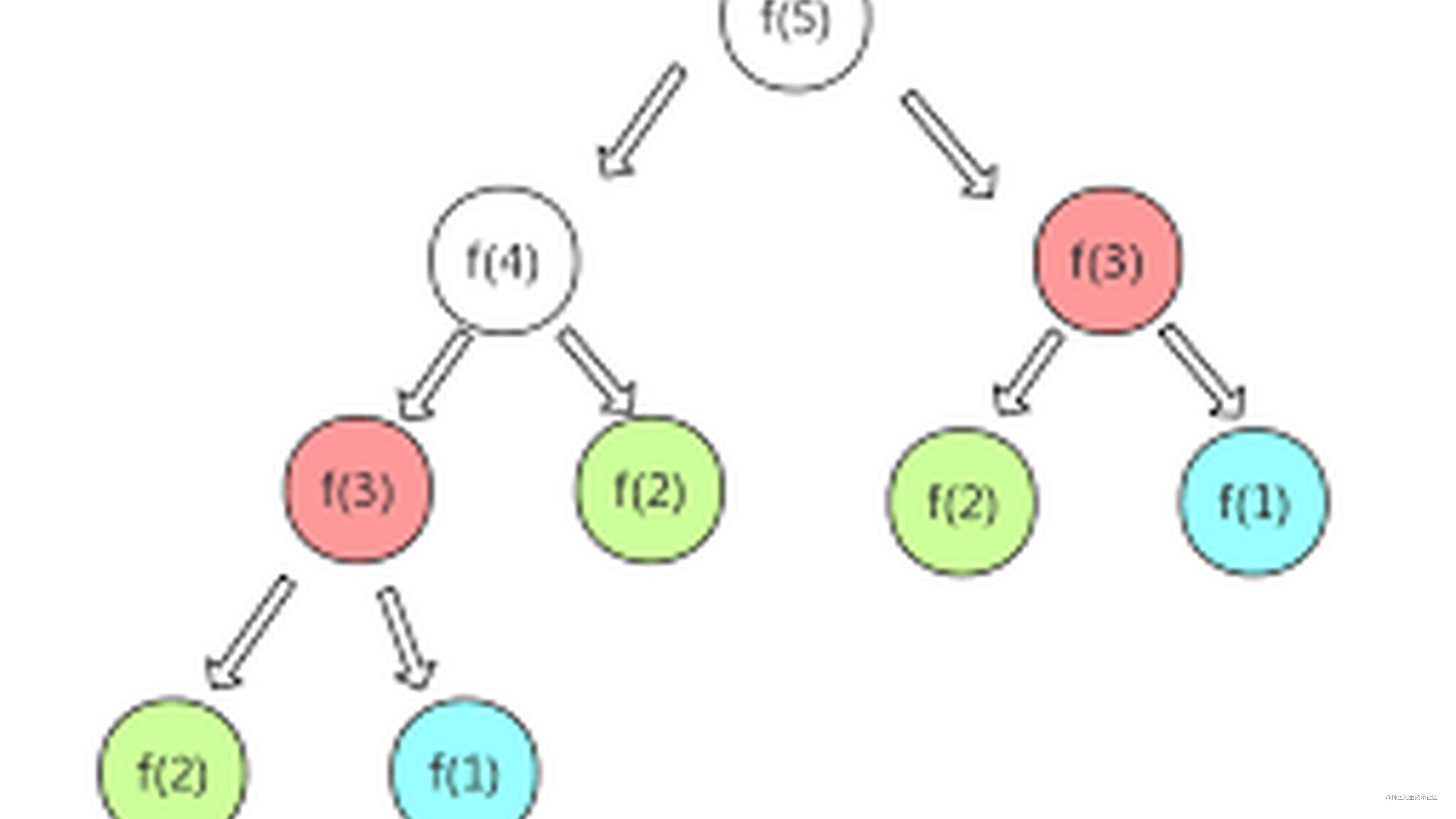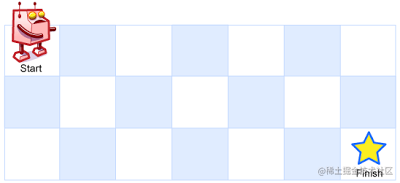# 聊一聊前端算法面试——递归### 写在前面

1. 假如楼梯有n个台阶，每次可以走1个或2个台阶，请问走完这n个台阶有几种走法(动态规划实现)❓
2. 如下图所示：一个机器人位于一个 m x n 网格的左上角 （起始点在下图中标记为“Start” ）。机器人每次只能向下或者向右移动一步。机器人试图达到网格的右下角（在下图中标记为“Finish”）。现在考虑网格中有障碍物。那么从左上角到右下角将会有多少条不同的路径❓1. 在M件物品里❓

### 如何编写递归代码❓

• 写出递归公式
• 找到终止条件

``````function sum(n) {
var total = 0
for (int i = 1; i <= n; i++) {
total = total + i
}
}

``````sum(n) = sum(n-1) + n
···
···
···
sum(100) = sum(99) + 100
sum(99) = sum(98) + 99
···
···
···
sum(5) = sum(4) + 5
sum(4) = sum(3) + 4
sum(3) = sum(2) + 3
sum(2) = sum(1) + 2
sum(1) = 1

``````function sum(n) {
return sum(n-1) + n
}

``````function sum(n) {
if( n ===1 ) return 1
return sum(n-1) + n
}

### 面试题1： 楼梯问题

``````1 1 1
1 2
2 1

``````function climbStairs(n) {
return climbStairs(n - 1) + climbStairs(n - 2)
}

``````climbStairs(n) = climbStairs(n-1) + climbStairs(n-2)
climbStairs(n-1) = climbStairs(n-2) + climbStairs(n-3)
···
···
···
climbStairs(5) = climbStairs(4) + climbStairs(3)
climbStairs(4) = climbStairs(3) + climbStairs(2)
climbStairs(3) = climbStairs(2) + climbStairs(1)
climbStairs(2) = 2
climbStairs(1) = 1

``````climbStairs(2) = 2
climbStairs(1) = 1

``````function climbStairs(n) {
if (n == 1) return 1
if (n == 2) return 2
return climbStairs(n-1) + climbStairs(n-2)
}

``````var calculated = []

function climbStairs(n) {

if(n == 1) {
return 1
}else if (n == 2) {
return 2
}else {
if(!calculated[n-1]){
calculated[n-1] = climbStairs(n-1)
}

if(!calculated[n-2]){
calculated[n-2] = climbStairs(n-2)
}
return calculated[n-1] + calculated[n-2]
}

}

### 面试题2：实现深拷贝

``````var deepCopy = function(obj) {
if (typeof obj !== 'object') return;
// // 根据obj的类型判断是新建一个数组还是对象
var newObj = obj instanceof Array ? [] : {};
for (var key in obj) {
if (obj.hasOwnProperty(key)) {
newObj[key] = typeof obj[key] === 'object' ? deepCopy(obj[key]) : obj[key];
}
}
return newObj;
}

### 面试题3：如何把数组拍平

``````const flatten = (arr) => {
let result = [];
arr.forEach((item, i, arr) => {
// 若为数组,递归调用 faltten,并将结果与result合并
if (Array.isArray(item)) {
result = result.concat(flatten(item));
} else {
result.push(arr[i])
}
})
return result;
};
const arr = [1, [2, [3, 4, 5]]];
console.log(flatten(arr)); // [1, 2, 3, 4, 5]

### 总结